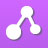cancel
Showing results for
Search instead for
Did you mean:New Contributor

## Basic Buffer Principles - Preparation and Use

This is a simple question about preparing a sodium acetate solution:

There are multiple ways to prepare a sodium acetate solution to a target pH and molarity. One can (a) take a calculated quantity of acetic acid and titrate with sodium hydroxide to target pH, or (b) one can take a calculated amount of sodium acetate and blend with a calculated amount of acetic acid with a fine pH adjustment if needed.

In protein chromatography (large scale downstream processing) I'm used to seeing (b) the more common approach, and have been under the assumption (a) is not an ideal way to create a buffer consistently at scale.  I'm having trouble though with the understanding of "why" that might be the case.  Any explanation would be appreciated.

PB